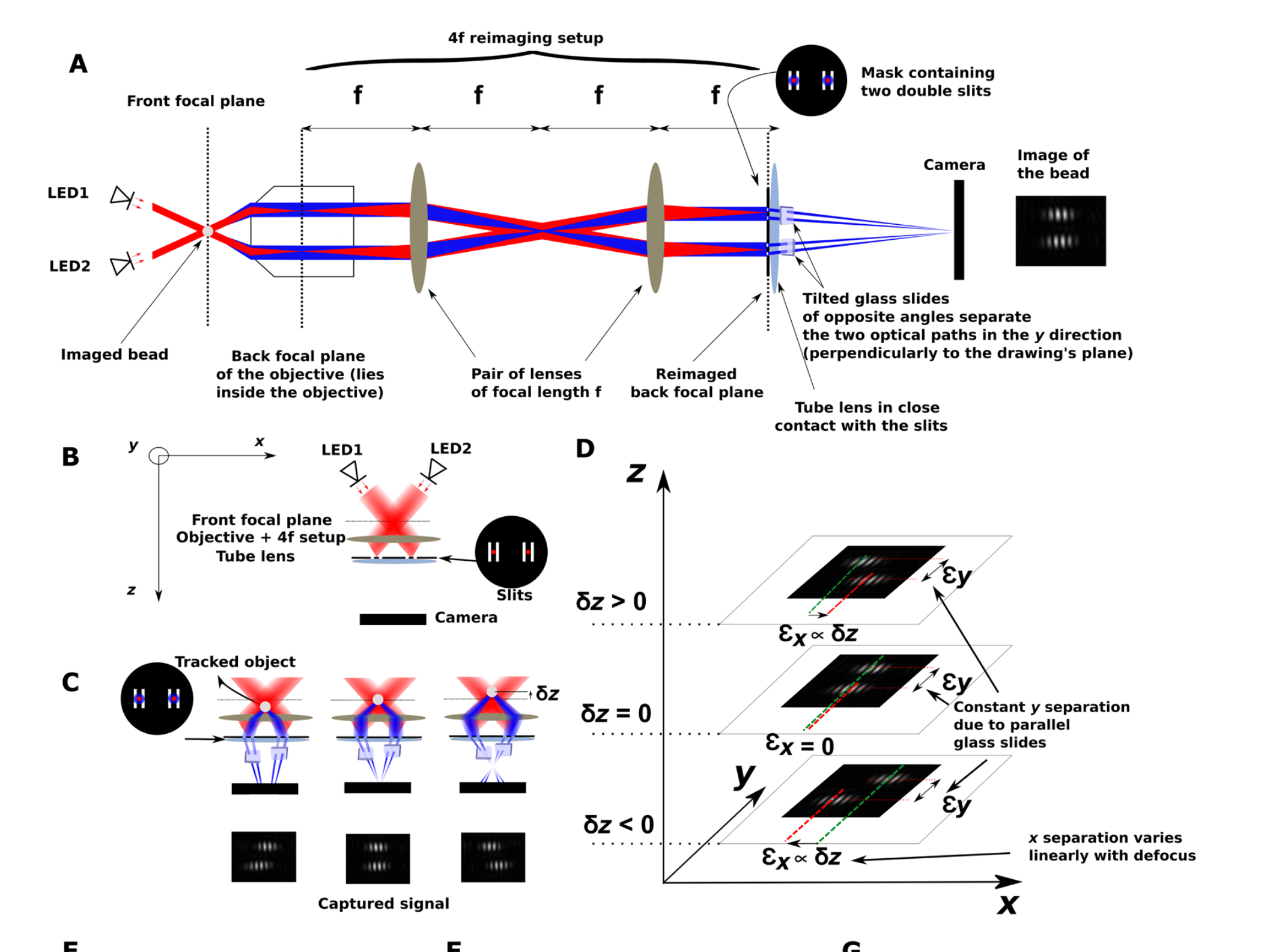# Literature/202310101212 stereo darkfield interferometry

First published:

Last Edited:

Number of edits:

One of the biggest issues with 3D nanoparticle tracking is that instruments lack sufficient resolution (both temporal and spatial). Stereo Darkfield Interferometry (SDI) solves some of the issues by using a combination of techniques in a "simple" to assemble microscope.By adding a combination of a dark-field microscope (in the image above using two superluminescent LED's) with a slit configuration (right at the tube-lens), with two wedges that allow to separate the stereoscopic interference pattern, they can achieve full 3D localization with better than $nm$ accuracy.

I find it very interesting that the noise for the localization is given by:

$$\sigma_i^2 > \frac{1}{g_i^2N}\frac{1}{\int \frac{f'(i)^2}{f(i)}di}$$

$g$ is related to the magnification, $N$ to the number of photons, but the interesting argument is the integral of the intensity profile ($f$). Since it depends on the derivative (squared!), the quicker the profile changes, the higher the precision.

Is perhaps these way of considering accuracy related to the way minflux works?

I also wonder if a method like this can be combined with a technique like SP-IRIS? In principle the interference pattern depends on the refractive index of the particle, which could be used for singe-shot sizing?